• ## 雅克比矩阵

千次阅读 2019-09-02 15:18:52
文章目录雅克比矩阵推导雅可比矩阵的代码实现 计算雅克比矩阵 雅克比矩阵推导 函数h(x)相对于x的导数，被称作雅可比式。 推到过程如下： 为了计算这个函数的导数，我们使用了商的求导规则。 给定一个函数z，它...
文章目录雅克比矩阵推导雅可比矩阵的代码实现
计算雅克比矩阵
雅克比矩阵推导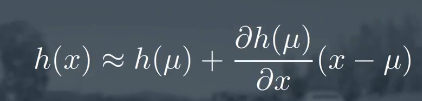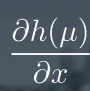函数h(x)相对于x的导数，被称作雅可比式。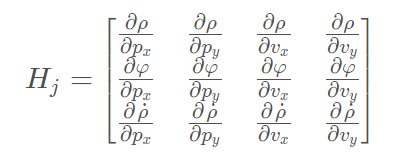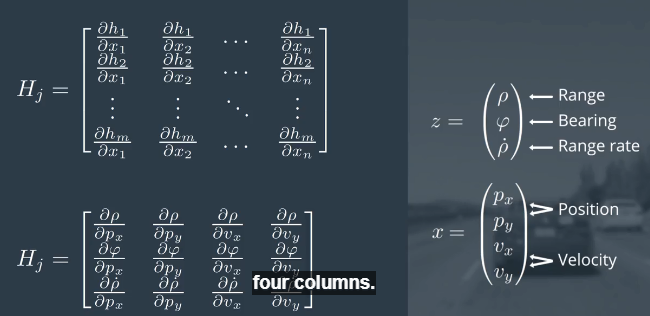推到过程如下：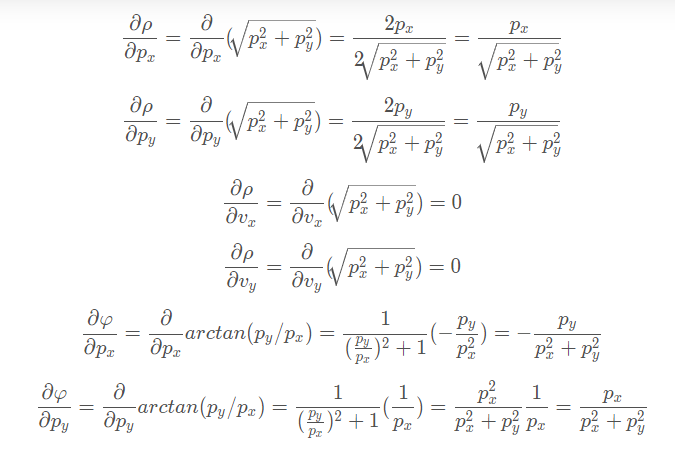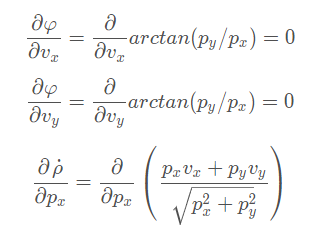为了计算这个函数的导数，我们使用了商的求导规则。
给定一个函数z，它是另外两个函数f和g的商：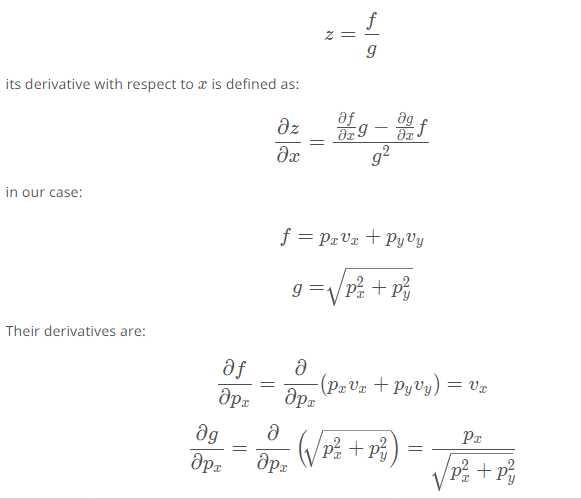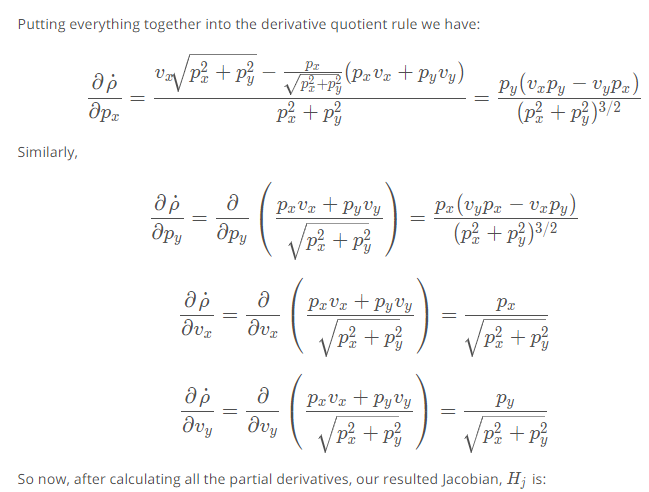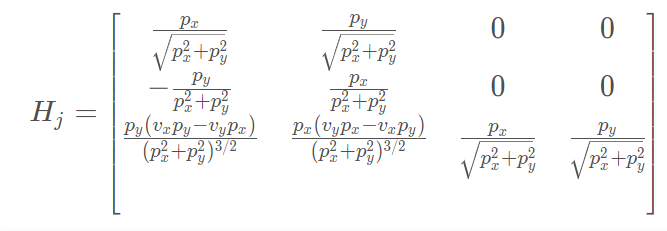注意：必须保证雅可比矩阵的除数（分母）不为零。
雅可比矩阵的代码实现
#include <iostream>
#include <vector>
#include "Dense"

using Eigen::MatrixXd;
using Eigen::VectorXd;
using std::cout;
using std::endl;

MatrixXd CalculateJacobian(const VectorXd& x_state);

int main() {
/**
* Compute the Jacobian Matrix
*/

// predicted state example
// px = 1, py = 2, vx = 0.2, vy = 0.4
VectorXd x_predicted(4);
x_predicted << 1, 2, 0.2, 0.4;

MatrixXd Hj = CalculateJacobian(x_predicted);

cout << "Hj:" << endl << Hj << endl;

return 0;
}

MatrixXd CalculateJacobian(const VectorXd& x_state) {

MatrixXd Hj(3,4);
// recover state parameters
float px = x_state(0);
float py = x_state(1);
float vx = x_state(2);
float vy = x_state(3);

// check division by zero
// if (fabs(c1) < 0.0001) {
//   cout << "CalculateJacobian () - Error - Division by Zero" << endl;
//   return Hj;
// }

// check division by zero
if (px != 0 and py != 0)
{
float dt_2 = px*px + py*py;
float dt_sqrt = sqrt(dt_2);
float dt_sqrt_3 = sqrt(dt_2*dt_2*dt_2);
// compute the Jacobian matrix
Hj << px/dt_sqrt, py/dt_sqrt, 0, 0,
-py/dt_2, px/dt_2, 0, 0,
py*(vx*py-vy*px)/dt_sqrt_3, px*(vy*px-vx*py)/dt_sqrt_3, px/dt_sqrt, py/dt_sqrt;

}
// compute the Jacobian matrix

return Hj;
}

运行结果如下：
Hj:
0.447214 0.894427        0        0
-0.4      0.2        0        0
0        0 0.447214 0.894427



展开全文• 雅克比矩阵平时经常会遇到，一直没怎么去好好理解。查询了网络大神的解答，自己做一个小小的记录。 雅克比矩阵最近遇到的场景是在微积分换元时，看到可以做一些变换。 其中求解雅克比矩阵行列式进行换元的方法...
雅克比矩阵平时经常会遇到，一直没怎么去好好理解。查询了网络大神的解答，自己做一个小小的记录。

雅克比矩阵最近遇到的场景是在微积分换元时，看到可以做一些变换。

其中求解雅克比矩阵行列式进行换元的方法此前也没有见到过。

在向量的微积分中，雅克比矩阵是一个一阶偏导数排列成的矩阵，其行列式是雅克比行列式。

在知乎上看到了相关的解答，解释了相关的含义。

在一维几何上，由于一维上度量dx可以反映任意自由度为1的变量的全部特征，所述一阶微分不变性。

可以想象在一个绳子上前进（一个维度），移动路程实际上只要一个变量即可确定。

2dt其实也就是对换元函数x=2t求了一阶导数，一元矩阵的行列式也就是本身的值吧

在二维的情况下，解答说明的是一种平面之间的投影关系

当我们使用 的时候，两个矢量之间的变换，实际上有 det(J)就是 这一组微分在 这一个平面上的投影。

额，网上作者有一个插图，我也借鉴过来，图是来自于彭家贵微分几何书籍，非数学专业不太了解。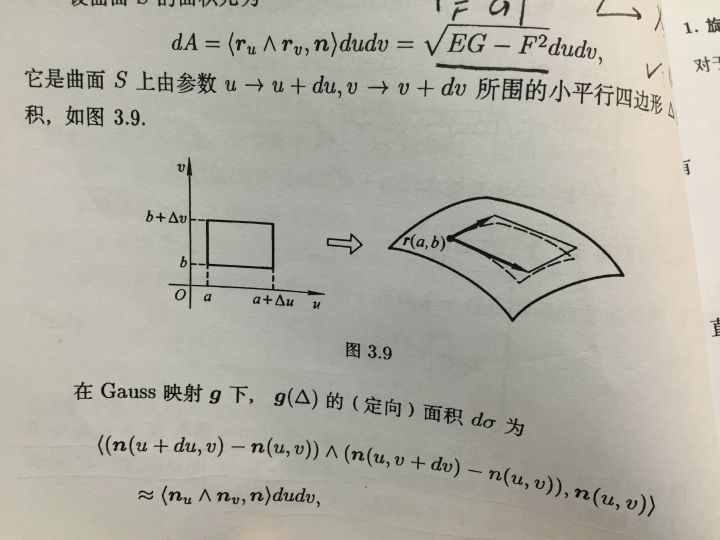可以看到任意一组非直线参数下的面积投影到平面正交参数上，可以写成雅克比矩阵的行列式（这里是雅克比矩阵行列式的平方开根号，[E,F;F,G]为雅克比矩阵乘以其转置）；再将另一组参数也投影一次，亦可以写成这个形式；这样就间接得到这两组参数相互投影的面积比值。

——————

理论说明

微分其实就是线性化，导数其实是线性空间之间的线性变换，雅克比矩阵本质上就是导数。

哈哈，如果这么说，积分换元要求原本元对新元的雅克比矩阵行列式就说得通了。

以下是更深层次的说明，

比如，映射 在x处的导数  就是 M 在 x 处的切空间  到 N 在 f(x) 处的切空间  之间的线性映射。

切空间都是矢量空间，都有基底，这个线性变换就是一个矩阵。

在欧式空间子空间的开集上，切空间就是某个, 实轴上的切空间就是R，曲面上的切空间是

这样一来，函数 的导数无非是切空间  到切空间   的线性变换，是一个1*1的雅克比矩阵，同构于一个实数。

因此雅克比矩阵实质上是切空间之间的基底之间的线性变换，因而积分中变换坐标时，会在前面乘以一个雅克比矩阵的行列式，行列式就是一个数值了，积分本身也是数值计算，而不是矢量计算吧，所以会是行列式。

———————

下面是最为常用的，雅克比计算方式

雅克比矩阵的重要在于它体现了一个可微分方程与给出点的最优线性逼近。雅克比矩阵类似于多元函数的导数。

定义

在代数几何中，代数曲线的雅克比行列式表示雅克比族：伴随该曲线的一个代数群，曲线可以嵌入其中。

假设某F：到 是一个从n维欧式空间映射到m维欧氏空间的函数，这个函数有m个实函数组成。

雅克比我常常见到是解决一个方程组问题时，需要求解的对象，方程组和这里说的m个实函数是一样的。

这些函数的偏导数，可以组成一个m行n列的矩阵，就是雅克比矩阵。

此矩阵用符号表示为：

或者

yi (i=1,2,...m)这个矩阵的第i行是由梯度函数的转置表示的

如果p是中的一点，F在p点可微分，相当于高等微积分， 是这点的导数。在此情况下，这个线性映射，

即是F在点P附近的最优线性逼近，也就是当x靠近p点时，有一下关系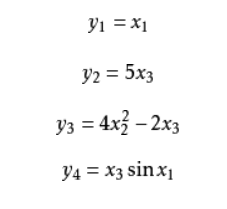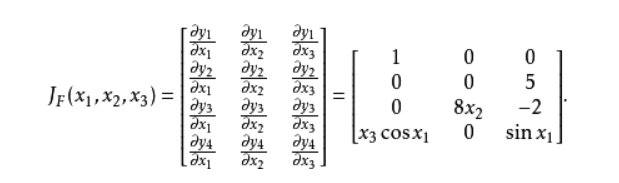____________

雅克比矩阵  是一个矩阵值函数，是多元向量值函数（vector-valued multivariate funciton)，多元就是n个维度的自变量，向量值函数就是映射的结果是一个向量，m维。

它是多元向量值函数 泰勒展开的一阶项系数。

一阶项 中的乘法是矩阵乘法。高阶项中的乘法涉及到张量乘法，符号复杂，哈哈，看到这个解答就知道自己了解实在太浅。

可以对比，当m=1时候，f退化为多元标量值函数（scalar-valued multivaiate function) ，这个时候雅克比矩阵退化为行向量，是梯度的转置

这时候一阶项中的乘法是矩阵乘法，

二阶项中的乘法也是矩阵乘法。

这里矩阵值函数 被称为海森矩阵（Hessian matrix)。类似地，高阶项中的乘法涉及到张量乘法。。。

个人理解，上面两处的泰勒展开无论是多元向量值函数，还是多元标量值函数都是一样的。

——————————

摘抄自博客和知乎上的大牛

雅克比矩阵 - feifanren - 博客园 (cnblogs.com)


展开全文• 文章目录一、scara机器人雅克比矩阵1、雅克比矩阵推导2、机器人末端速度与关节速度、末端加速度与关节加速度之间相互转换(1)、已知关节速度，计算末端速度(2)、已知关节加速度，计算末端加速度(3)、已知末端速度，...
文章目录一、scara机器人雅克比矩阵1、雅克比矩阵推导2、机器人末端速度与关节速度、末端加速度与关节加速度之间相互转换(1)、已知关节速度，计算末端速度(2)、已知关节加速度，计算末端加速度(3)、已知末端速度，计算关节速度(4)、已知末端加速度，计算关节加速度二、雅克比矩阵验证1、点到点运动2、直线运动三、MATLAB代码
一、scara机器人雅克比矩阵
1、雅克比矩阵推导
scara机器人的运动学正解为：
$\begin{cases} x=L_1cos\theta_1+L_2cos(\theta_1+\theta_2) \\ y=L_1sin\theta_1+L_2sin(\theta_1+\theta_2) \\ z=\frac{\theta_3s}{2\pi} \\ c=\theta_1+ \theta_2+ \theta_4 \tag 1 \end{cases}$
参考我另一篇博文:scara机器人运动学正逆解。
上式两边对时间$t$求导，得：
$\begin{cases} v_x=-L_1sin\theta_1\dot{\theta_1}-L_2sin(\theta_1+\theta_2)(\dot{\theta_1} + \dot{\theta_2}) \\ v_y=L_1cos\theta_1\dot{\theta_1}+L_2cos(\theta_1+\theta_2) (\dot{\theta_1} + \dot{\theta_2}) \\ v_z=\frac{\dot{\theta_3}s}{2\pi} \\ v_c=\dot{\theta_1}+ \dot{\theta_2}+ \dot{\theta_4} \tag 2 \end{cases}$
上式写成矩阵形式，得：
$\left[ \begin{matrix} v_x \\ v_y \\ v_z \\ v_c \end{matrix} \right] = J \left[ \begin{matrix} \dot{\theta_1} \\ \dot{\theta_2} \\ \dot{\theta_3} \\ \dot{\theta_4} \end{matrix} \right] \tag{3}$
其中$J$为雅克比矩阵：
$J=\left[ \begin{matrix} -L_1sin\theta_1-L_2sin(\theta_1+\theta_2) & -L_2sin(\theta_1+\theta_2) & 0 & 0\\ L_1cos\theta_1+L_2cos(\theta_1+\theta_2) & L_2cos(\theta_1+\theta_2) & 0 & 0 \\ 0 & 0 & s/(2\pi) & 0 \\ 1 & 1 & 0 & 1 \end{matrix} \right] \tag{4}$
由式(3)可知，雅克比矩阵是机器人末端速度与关节速度的桥梁，是两者间的映射关系。雅克比矩阵的行列式为：
\begin{aligned} |J|&=\left| \begin{matrix} -L_1sin\theta_1-L_2sin(\theta_1+\theta_2) & -L_2sin(\theta_1+\theta_2) & 0 & 0\\ L_1cos\theta_1+L_2cos(\theta_1+\theta_2) & L_2cos(\theta_1+\theta_2) & 0 & 0 \\ 0 & 0 & s/(2\pi) & 0 \\ 1 & 1 & 0 & 1 \end{matrix} \right| \\ &= \frac{L_1L_2s}{2\pi}sin{\theta_2} \\ \tag{5} \end{aligned}
当$sin{\theta_2}=0$时，$|J|=0$，雅克比矩阵不可逆，此时机器人处于奇异位置。在奇异位置，无法通过机器人末端速度和雅克比矩阵计算得到机器人关节速度。或者说，在奇异位置，通过机器人末端速度和雅克比矩阵计算得到的机器人关节速度为无穷大，这在实际物理世界是不可能的。因此，机器人笛卡尔空间插补运动(直线、圆弧、样条插补等)一定要考虑并避开奇异位置。
2、机器人末端速度与关节速度、末端加速度与关节加速度之间相互转换
(1)、已知关节速度，计算末端速度
已知机器人在工作空间内任意位置(即使是奇异位置，即$sin{\theta_2}=0$)的关节位置和关节速度，通过雅克比矩阵，可以根据式(3)直接求得。
(2)、已知关节加速度，计算末端加速度
式(3)两边对时间t求导，得：
$\left[ \begin{matrix} a_x \\ a_y \\ a_z \\ a_c \end{matrix} \right] = \dot{J} \left[ \begin{matrix} \dot{\theta_1} \\ \dot{\theta_2} \\ \dot{\theta_3} \\ \dot{\theta_4} \end{matrix} \right] +J\left[ \begin{matrix} \ddot{\theta_1} \\ \ddot{\theta_2} \\ \ddot{\theta_3} \\ \ddot{\theta_4} \end{matrix} \right] \tag{6}$
其中：
$\dot{J}=\left[ \begin{matrix} -L_1cos\theta_1\dot{\theta_1}-L_2cos(\theta_1+\theta_2) (\dot{\theta_1}+\dot{\theta_2}) & -L_2cos(\theta_1+\theta_2)(\dot{\theta_1}+\dot{\theta_2}) & 0 & 0\\ -L_1sin\theta_1\dot{\theta_1}-L_2sin(\theta_1+\theta_2)(\dot{\theta_1}+\dot{\theta_2}) & -L_2sin(\theta_1+\theta_2)(\dot{\theta_1}+\dot{\theta_2}) & 0 & 0 \\ 0 & 0 & 0 & 0 \\ 0 & 0 & 0 & 0 \end{matrix} \right] \tag{7}$
(3)、已知末端速度，计算关节速度
当机器人不在奇异位置时，根据式(3)，关节速度如下计算：
$\left[ \begin{matrix} \dot{\theta_1} \\ \dot{\theta_2} \\ \dot{\theta_3} \\ \dot{\theta_4} \end{matrix} \right] = J^{-1}\left[ \begin{matrix} v_x \\ v_y \\ v_z \\ v_c \end{matrix} \right] \tag{8}$
由于3,4轴关节速度很容易根据式(2)单独求得：
$\begin{cases} \dot{\theta_3}=2\pi v_z / s \\ \dot{\theta_4}=v_c-\dot{\theta_1}- \dot{\theta_2} \\ \tag {9} \end{cases}$
只考虑1,2轴关节速度的计算显得更加简单，计算量也更小。令：
$\begin{cases} s_1=sin\theta_1 \\ s_{12}=sin(\theta_1+\theta_2) \\ c_1=cos\theta_1 \\ c_{12}=cos(\theta_1+\theta_2) \tag {10} \end{cases}$
$\begin{cases} a_{12}=-L_2s_{12} \\ a_{11}=-L_1s_1+a_{12} \\ b_1=v_x \\ a_{22}=L_2c_{12} \\ a_{21}=L_1c_1+a_{22} \\ b_2=v_y \\ \tag {11} \end{cases}$
式(2)转化为关于$\dot{\theta_1}$和$\dot{\theta_2}$的二元一次方程组：
$\begin{cases} a_{11}\dot{\theta_1}+a_{12}\dot{\theta_2}=b_1 \\ a_{21}\dot{\theta_1}+a_{22}\dot{\theta_2}=b_2 \\ \tag {12} \end{cases}$
上式解得：
$\begin{cases} \dot{\theta_1}=-(a_{12}b_2 - a_{22}b_1)/(a_{11}a_{22} - a_{12}a_{21}) \\ \dot{\theta_2}=(a_{11}b_2 - a_{21}b_1)/(a_{11}a_{22} - a_{12}a_{21}) \\ \tag {13} \end{cases}$
(4)、已知末端加速度，计算关节加速度
当机器人不在奇异位置时，根据式(6)，关节加速度如下计算：
$\left[ \begin{matrix} \ddot{\theta_1} \\ \ddot{\theta_2} \\ \ddot{\theta_3} \\ \ddot{\theta_4} \end{matrix} \right]=J^{-1}\left( \left[ \begin{matrix} a_x \\ a_y \\ a_z \\ a_c \end{matrix} \right] -\dot{J} \left[ \begin{matrix} \dot{\theta_1} \\ \dot{\theta_2} \\ \dot{\theta_3} \\ \dot{\theta_4} \end{matrix} \right] \right) \tag{14}$
由于3,4轴关节加速度很容易根据式(9)单独求得：
$\begin{cases} \ddot{\theta_3}=2\pi a_z / s \\ \ddot{\theta_4}=a_c-\ddot{\theta_1}- \ddot{\theta_2} \\ \tag {15} \end{cases}$
只考虑1,2轴关节加速度的计算显得更加简单，计算量也更小。式(2)两边对时间t求导，得：
$\begin{cases} a_x=-L_1(cos\theta_1\dot{\theta_1}^2 + sin\theta_1\ddot{\theta_1})-L_2[cos(\theta_1+\theta_2)(\dot{\theta_1} + \dot{\theta_2})^2 \\ \ \ \ \ \ \ \ \ \ + sin(\theta_1+\theta_2)(\ddot{\theta_1} + \ddot{\theta_2})] \\ a_y=L_1(-sin\theta_1\dot{\theta_1}^2+cos\theta_1\ddot{\theta_1})+L_2[-sin(\theta_1+\theta_2) (\dot{\theta_1} + \dot{\theta_2})^2 \\ \ \ \ \ \ \ \ \ \ +cos(\theta_1+\theta_2) (\ddot{\theta_1} + \ddot{\theta_2}) ]\\ \tag {16} \end{cases}$
令：
$\begin{cases} d_1=a_x+L_1c_1\dot{\theta_1}^2+L_2c_{12}(\dot{\theta_1} + \dot{\theta_2})^2 \\ d_2=a_y +L_1s_1\dot{\theta_1}^2+L_2s_{12}(\dot{\theta_1} + \dot{\theta_2})^2\\ \tag {17} \end{cases}$
式(16)转化为关于$\ddot{\theta_1}$和$\ddot{\theta_2}$的二元一次方程组：
$\begin{cases} a_{11}\ddot{\theta_1}+a_{12}\ddot{\theta_2}=d_1 \\ a_{21}\ddot{\theta_1}+a_{22}\ddot{\theta_2}=d_2 \\ \tag {18} \end{cases}$
上式解得：
$\begin{cases} \ddot{\theta_1}=-(a_{12}d_2 - a_{22}d_1)/(a_{11}a_{22} - a_{12}a_{21}) \\ \ddot{\theta_2}=(a_{11}d_2 - a_{21}d_1)/(a_{11}a_{22} - a_{12}a_{21}) \\ \tag {19} \end{cases}$
二、雅克比矩阵验证
1、点到点运动
(1)在$[-\pi, \pi]$中随机生成4个关节角度，生成点到点运动起点与终点（模拟示教过程）
(2)速度规划(详见另一篇博文：归一化5次多项式速度规划)
(3)ptp插补得到关节位置、速度、加速度
(4)运动学正解得到笛卡尔位置(x,y,z,c)，并作差分运算，估计笛卡尔分轴速度、加速度
(5)利用雅克比矩阵计算笛卡尔分轴速度、加速度
(6)绘图比较(4)与(5)的差异，验证雅克比矩阵正确性
2、直线运动
(1)给定直线运动起点与终点，手系handcoor，标志位flagJ1和flagJ2（模拟示教过程）
(2)速度规划(详见另一篇博文：归一化5次多项式速度规划)
(3)直线插补得到笛卡尔位置、速度、加速度
(4)运动学逆解得到关节位置，并作差分运算，估计关节速度、加速度
(5)利用雅克比矩阵计算关节速度、加速度
(6)绘图比较(4)与(5)的差异，验证雅克比矩阵正确性
三、MATLAB代码
%{
Function: scara_forward_kinematics
Description: scara机器人运动学正解
Author: Marc Pony(marc_pony@163.com)
%}
function cartesianPos = scara_forward_kinematics(L1, L2, screw, jointPos)
theta1 = jointPos(1);
theta2 = jointPos(2);
theta3 = jointPos(3);
theta4 = jointPos(4);
x = L1 * cos(theta1) + L2 * cos(theta1 + theta2);
y = L1 * sin(theta1) + L2 * sin(theta1 + theta2);
z = theta3 * screw / (2 * pi);
c = theta1 + theta2 + theta4;
cartesianPos = [x; y; z; c];
end

%{
Function: scara_inverse_kinematics
Description: scara机器人运动学逆解
手系handcoor，标志位flagJ1与flagJ2
Author: Marc Pony(marc_pony@163.com)
%}
function jointPos = scara_inverse_kinematics(L1, L2, screw, cartesianPos, handcoor, flagJ1, flagJ2)
x = cartesianPos(1);
y = cartesianPos(2);
z = cartesianPos(3);
c = cartesianPos(4);
jointPos = zeros(4, 1);

calculateError = 1.0e-8;
c2 = (x^2 + y^2 - L1^2 -L2^2) / (2.0 * L1 * L2);%若(x,y)在工作空间里，则c2必在[-1,1]里，但由于计算精度，c2的绝对值可能稍微大于1
temp = 1.0 - c2^2;

if temp < 0.0
if temp > -calculateError
temp = 0.0;
else
error('区域不可到达');
end
end
if handcoor == 0    %left handcoor
jointPos(2) = atan2(-sqrt(temp), c2);
else                %right handcoor
jointPos(2) = atan2(sqrt(temp), c2);
end
s2 = sin(jointPos(2));
jointPos(1) = atan2(y, x) - atan2(L2 * s2, L1 + L2 * c2);
jointPos(3) = 2.0 * pi * z / screw;

if jointPos(1) <= -pi
jointPos(1) = jointPos(1) + 2.0*pi;
end
if jointPos(1) >= pi
jointPos(1) = jointPos(1) - 2.0*pi;
end

if flagJ1 == 1
if jointPos(1) >= 0.0
jointPos(1) = jointPos(1) - 2.0*pi;
else
jointPos(1) = jointPos(1) + 2.0*pi;
end
end

if flagJ2 == 1
if jointPos(2) >= 0.0
jointPos(2) = jointPos(2) - 2.0*pi;
else
jointPos(2) = jointPos(2) + 2.0*pi;
end
end
jointPos(4) = c - jointPos(1) - jointPos(2);
end

clc;
clear;
close all;
syms L1 L2 theta1 theta2 s real
syms dtheta1 dtheta2 dtheta3 dtheta4 ddtheta1 ddtheta2 ddtheta3 ddtheta4 real
syms vx vy vz vc ax ay az ac real;

J = [-L1*sin(theta1) - L2*sin(theta1 + theta2),  -L2*sin(theta1 + theta2), 0, 0
L1*cos(theta1) + L2*cos(theta1 + theta2),  L2*cos(theta1 + theta2), 0, 0
0, 0, s/(2*pi), 0
1, 1, 0, 1];
detJ = simplify(det(J))

dJ = [-L1*cos(theta1)*dtheta1 - L2*cos(theta1 + theta2)*(dtheta1 + dtheta2), -L2*cos(theta1 + theta2)*(dtheta1 + dtheta2), 0, 0
-L1*sin(theta1)*dtheta1 - L2*sin(theta1 + theta2)*(dtheta1 + dtheta2), -L2*sin(theta1 + theta2)*(dtheta1 + dtheta2), 0, 0
0, 0, 0, 0
0, 0, 0, 0];

cartesianVel = simplify(J * [dtheta1; dtheta2; dtheta3; dtheta4])
cartesianAcc = simplify(dJ * [dtheta1; dtheta2; dtheta3; dtheta4] + J * [ddtheta1; ddtheta2; ddtheta3; ddtheta4])
jointVel = simplify(J \ [vx; vy; vz; vc])
jointAcc = simplify(J \ ([ax; ay; az; ac] - dJ * jointVel))

%% 输入参数
len1 = 200; %mm
len2 = 200; %mm
screw = 20; %mm
speedJ = 200; %°/s
accJ = 1200; %°/s^2
linearSpeed = 100; %mm/s
linearAcc = 800;   %mm/s^2
orientationSpeed = 200; %°/s
orientationAcc = 600;   %°/s^2
dt = 0.001; %s

%% 点到点运动, 验证雅克比矩阵正确性
%(1)在[-pi, pi]中随机生成4个关节角度，生成点到点运动起点与终点（模拟示教过程）
%(2)速度规划
%(3)ptp插补得到关节位置、速度、加速度
%(4)运动学正解得到笛卡尔位置(x,y,z,c)，并作差分运算，估计笛卡尔分轴速度、加速度
%(5)利用雅克比矩阵计算笛卡尔分轴速度、加速度
%(6)绘图比较(4)与(5)的差异，验证雅克比矩阵正确性
theta = (-pi + 2.0*pi*rand(2, 4))*180.0/pi;
L = max(abs(diff(theta)));
T = max([1.875 * L / speedJ, sqrt(5.7735 * L / accJ)]);

t = (0 : dt : T)';
u = t / T;
if abs(t(end) - T) > 1.0e-8
t = [t; T];
u = [u; 1];
end
u = t / T;
s = 10*u.^3 - 15*u.^4 + 6*u.^5;
ds = 30*u.^2 -60*u.^3 + 30*u.^4;
dds = 60*u - 180*u.^2 + 120*u.^3;

len = L * s;
vel = (L / T) * ds;
acc = (L / T^2) * dds;
rate = diff(theta) / L;
jointPos = zeros(length(t), 4);
jointVel = zeros(length(t), 4);
jointAcc = zeros(length(t), 4);
cartesianPos = zeros(length(t), 4);
cartesianVel = zeros(length(t), 4);
cartesianAcc = zeros(length(t), 4);
for i = 1 : length(t)
jointPos(i, :) = (theta(1, :) + len(i) * rate)*pi/180.0;
jointVel(i, :) = (vel(i) * rate)*pi/180.0;
jointAcc(i, :) = (acc(i) * rate)*pi/180.0;
cartesianPos(i, :) = scara_forward_kinematics(len1, len2, screw, jointPos(i, :));
cartesianPos(i, 4) = cartesianPos(i, 4)*180.0/pi;

theta1 = jointPos(i, 1);
theta2 = jointPos(i, 2);
J = [-len1*sin(theta1) - len2*sin(theta1 + theta2),  -len2*sin(theta1 + theta2), 0, 0
len1*cos(theta1) + len2*cos(theta1 + theta2),  len2*cos(theta1 + theta2), 0, 0
0, 0, screw/(2*pi), 0
1, 1, 0, 1];

dtheta1 = jointVel(i, 1);
dtheta2 = jointVel(i, 2);
dtheta3 = jointVel(i, 3);
dtheta4 = jointVel(i, 4);
ddtheta1 = jointAcc(i, 1);
ddtheta2 = jointAcc(i, 2);
ddtheta3 = jointAcc(i, 3);
ddtheta4 = jointAcc(i, 4);

dJ = [-len1*cos(theta1)*dtheta1 - len2*cos(theta1 + theta2)*(dtheta1 + dtheta2), -len2*cos(theta1 + theta2)*(dtheta1 + dtheta2), 0, 0
-len1*sin(theta1)*dtheta1 - len2*sin(theta1 + theta2)*(dtheta1 + dtheta2), -len2*sin(theta1 + theta2)*(dtheta1 + dtheta2), 0, 0
0, 0, 0, 0
0, 0, 0, 0];

cartesianVel(i, :) = (J * [dtheta1; dtheta2; dtheta3; dtheta4])';
cartesianAcc(i, :) = (dJ * [dtheta1; dtheta2; dtheta3; dtheta4] + J * [ddtheta1; ddtheta2; ddtheta3; ddtheta4])';
cartesianVel(i, 4) = cartesianVel(i, 4)*180.0/pi;
cartesianAcc(i, 4) = cartesianAcc(i, 4)*180.0/pi;
end
cartesianVel2 = diff(cartesianPos) ./ diff(t);
cartesianAcc2 = diff(cartesianVel2) ./ diff(t(2:end));

figure(1)
set(gcf, 'color','w');
subplot(2,2,1)
plot(t, cartesianVel(:, 1), '--r')
hold on
plot(t(2:end), cartesianVel2(:, 1), 'b')
xlabel('t/s');
ylabel('v_x/ mm/s');
subplot(2,2,2)
plot(t, cartesianVel(:, 2), '--r')
hold on
plot(t(2:end), cartesianVel2(:, 2), 'b')
xlabel('t/s');
ylabel('v_y/ mm/s');
legend('通过雅克比矩阵换算','通过差分换算');
subplot(2,2,3)
plot(t, cartesianVel(:, 3), '--r')
hold on
plot(t(2:end), cartesianVel2(:, 3), 'b')
xlabel('t/s');
ylabel('v_z/ mm/s');
subplot(2,2,4)
plot(t, cartesianVel(:, 4), '--r')
hold on
plot(t(2:end), cartesianVel2(:, 4), 'b')
xlabel('t/s');
ylabel('v_c/ °/s');

figure(2)
set(gcf, 'color','w');
subplot(2,2,1)
plot(t, cartesianAcc(:, 1), '--r')
hold on
plot(t(3:end), cartesianAcc2(:, 1), 'b')
xlabel('t/s');
ylabel('a_x/ mm/s^2');
subplot(2,2,2)
plot(t, cartesianAcc(:, 2), '--r')
hold on
plot(t(3:end), cartesianAcc2(:, 2), 'b')
xlabel('t/s');
ylabel('a_y/ mm/s^2');
legend('通过雅克比矩阵换算','通过差分换算');
subplot(2,2,3)
plot(t, cartesianAcc(:, 3), '--r')
hold on
plot(t(3:end), cartesianAcc2(:, 3), 'b')
xlabel('t/s');
ylabel('a_z/ mm/s^2');
subplot(2,2,4)
plot(t, cartesianAcc(:, 4), '--r')
hold on
plot(t(3:end), cartesianAcc2(:, 4), 'b')
xlabel('t/s');
ylabel('a_c/ °/s^2');

%% 直线运动, 验证雅克比矩阵正确性
%(1)给定直线运动起点与终点，手系handcoor，标志位flagJ1和flagJ2（模拟示教过程）
%(2)速度规划
%(3)直线插补得到笛卡尔位置、速度、加速度
%(4)运动学逆解得到关节位置，并作差分运算，估计关节速度、加速度
%(5)利用雅克比矩阵计算关节速度、加速度
%(6)绘图比较(4)与(5)的差异，验证雅克比矩阵正确性
handcoor = 0;
flagJ1 = 0;
flagJ2 = 0;
pos = [200 100 0 60
250 200 20 80]; %mm °
x1 = pos(1, 1);
y1 = pos(1, 2);
z1 = pos(1, 3);
cc1 = pos(1, 4);
x2 = pos(2, 1);
y2 = pos(2, 2);
z2 = pos(2, 3);
cc2 = pos(2, 4);

L = sqrt((x2 - x1)^2 + (y2 - y1)^2 + (z2 - z1)^2);
C = abs(cc2 - cc1);
T = max([1.875 * L / linearSpeed, 1.875 * C / orientationSpeed, ...
sqrt(5.7735 * L / linearAcc), sqrt(5.7735 * C / orientationAcc)]);

t = (0 : dt : T)';
u = t / T;
if abs(t(end) - T) > 1.0e-8
t = [t; T];
u = [u; 1];
end
u = t / T;
s = 10*u.^3 - 15*u.^4 + 6*u.^5;
ds = 30*u.^2 -60*u.^3 + 30*u.^4;
dds = 60*u - 180*u.^2 + 120*u.^3;

len = L * s;
vel = (L / T) * ds;
acc = (L / T^2) * dds;
cartesianPos = zeros(length(t), 4);
cartesianVel = zeros(length(t), 4);
cartesianAcc = zeros(length(t), 4);
jointPos = zeros(length(t), 4);
jointVel = zeros(length(t), 4);
jointAcc = zeros(length(t), 4);
rate = [x2 - x1, y2 - y1, z2 - z1, cc2 - cc1] / L;
for i = 1 : length(t)

cartesianPos(i, :) = [x1, y1, z1, cc1] + len(i) * rate;
cartesianVel(i, :) = vel(i) * rate;
cartesianAcc(i, :) = acc(i) * rate;

cartesianPos(i, 4) = cartesianPos(i, 4)*pi/180.0;
jointPos(i, :) = scara_inverse_kinematics(len1, len2, screw, cartesianPos(i, :), handcoor, flagJ1, flagJ2);

theta1 = jointPos(i, 1);
theta2 = jointPos(i, 2);
s1 = sin(theta1);
s12 = sin(theta1 + theta2);
c1 = cos(theta1);
c12 = cos(theta1 + theta2);

vx = cartesianVel(i, 1);
vy = cartesianVel(i, 2);
vz = cartesianVel(i, 3);
vc = cartesianVel(i, 4)*pi/180.0;

a12 = -len2*s12;
a11 = -len1*s1 + a12;
b1 = vx;
a22 = len2*c12;
a21 = len1*c1 + a22;
b2 = vy;
temp = a11*a22 - a12*a21;

dtheta1 = -(a12*b2 - a22*b1)/temp;
dtheta2 = (a11*b2 - a21*b1)/temp;
dtheta3 = 2*pi*vz/screw;
dtheta4 = vc - dtheta1 - dtheta2;
jointVel(i, :) = [dtheta1, dtheta2, dtheta3, dtheta4];

ax = cartesianAcc(i, 1);
ay = cartesianAcc(i, 2);
az = cartesianAcc(i, 3);
ac = cartesianAcc(i, 4)*pi/180.0;

d1 = ax + len1*c1*dtheta1^2 + len2*c12*(dtheta1 + dtheta2)^2;
d2 = ay + len1*s1*dtheta1^2 + len2*s12*(dtheta1 + dtheta2)^2;
ddtheta1 = -(a12*d2 - a22*d1)/temp;
ddtheta2 = (a11*d2 - a21*d1)/temp;
ddtheta3 = 2*pi*az/screw;
ddtheta4 = ac - ddtheta1 - ddtheta2;

jointAcc(i, :) = [ddtheta1, ddtheta2, ddtheta3, ddtheta4];
end
jointVel2 = diff(jointPos) ./ diff(t);
jointAcc2 = diff(jointVel2) ./ diff(t(2:end));

figure(3)
set(gcf, 'color','w');
subplot(2,2,1)
plot(t, jointVel(:, 1)*180.0/pi, '--r')
hold on
plot(t(2:end), jointVel2(:, 1)*180.0/pi, 'b')
xlabel('t/s');
ylabel('vj1/ °/s');
subplot(2,2,2)
plot(t, jointVel(:, 2)*180.0/pi, '--r')
hold on
plot(t(2:end), jointVel2(:, 2)*180.0/pi, 'b')
xlabel('t/s');
ylabel('vj2/ °/s');
legend('通过雅克比矩阵换算','通过差分换算');
subplot(2,2,3)
plot(t, jointVel(:, 3)*180.0/pi, '--r')
hold on
plot(t(2:end), jointVel2(:, 3)*180.0/pi, 'b')
xlabel('t/s');
ylabel('vj3/ °/s');
subplot(2,2,4)
plot(t, jointVel(:, 4)*180.0/pi, '--r')
hold on
plot(t(2:end), jointVel2(:, 4)*180.0/pi, 'b')
xlabel('t/s');
ylabel('vj4/ °/s');

figure(4)
set(gcf, 'color','w');
subplot(2,2,1)
plot(t, jointAcc(:, 1), '--r')
hold on
plot(t(3:end), jointAcc2(:, 1), 'b')
xlabel('t/s');
ylabel('aj1/ °/s^2');
subplot(2,2,2)
plot(t, jointAcc(:, 2), '--r')
hold on
plot(t(3:end), jointAcc2(:, 2), 'b')
xlabel('t/s');
ylabel('aj2/ °/s^2');
legend('通过雅克比矩阵换算','通过差分换算');
subplot(2,2,3)
plot(t, jointAcc(:, 3), '--r')
hold on
plot(t(3:end), jointAcc2(:, 3), 'b')
xlabel('t/s');
ylabel('aj3/ °/s^2');
subplot(2,2,4)
plot(t, jointAcc(:, 4), '--r')
hold on
plot(t(3:end), jointAcc2(:, 4), 'b')
xlabel('t/s');
ylabel('aj4/ °/s^2');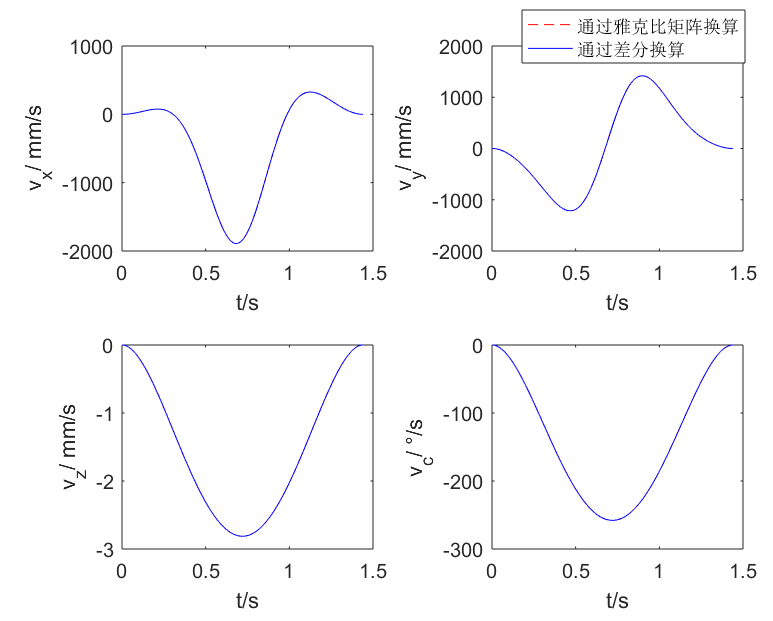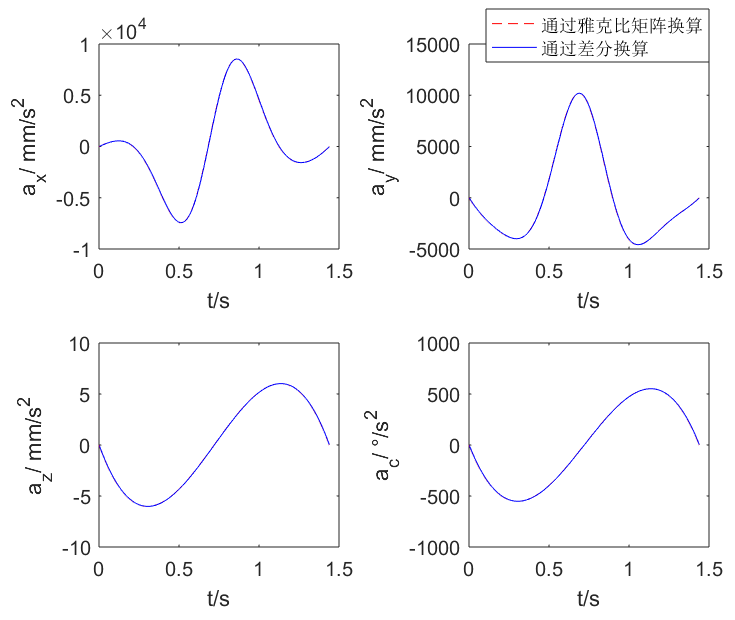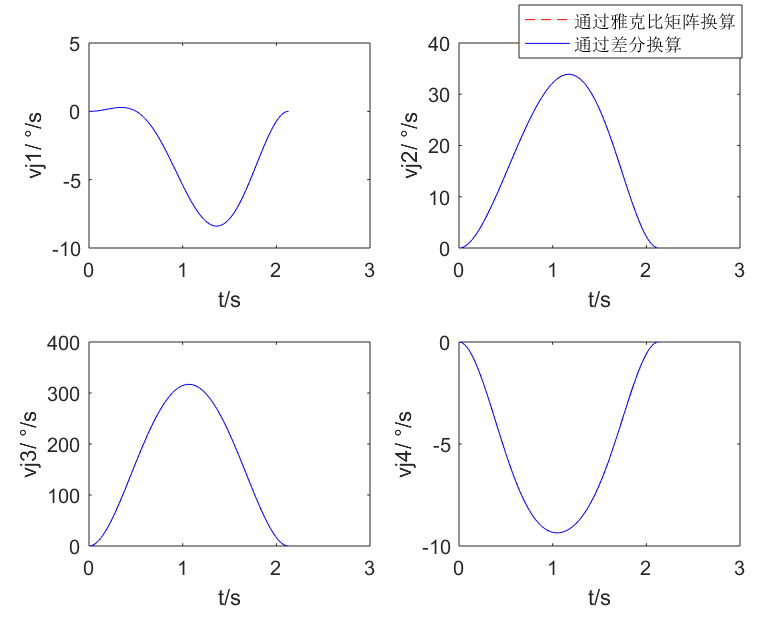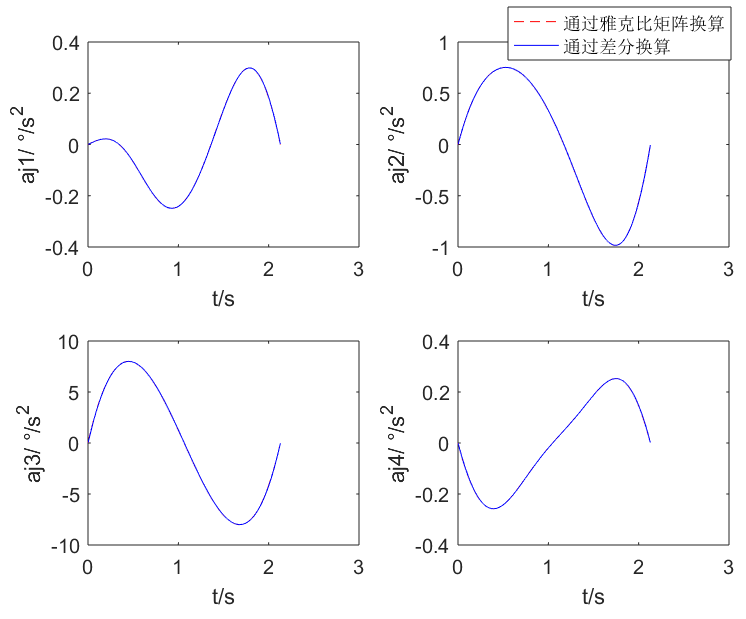展开全文• 雅克比矩阵就是一阶偏导数以一定方式排列地矩阵，这种行列式就是雅克比行列式。 雅克比矩阵重要性体现在一个可微方程与给出点的最优线性逼近。雅克比矩阵类似于多元函数的导数。 假设F: Rn→Rm是一个从欧式n维空间...
1、Jacobian
雅克比矩阵就是一阶偏导数以一定方式排列地矩阵，这种行列式就是雅克比行列式。
雅克比矩阵重要性体现在一个可微方程与给出点的最优线性逼近。雅克比矩阵类似于多元函数的导数。
假设F: Rn→Rm是一个从欧式n维空间转换到欧式m维空间的函数. 这个函数由m个实函数组成: y1(x1,…,xn), …, ym(x1,…,xn). 这些函数的偏导数(如果存在)可以组成一个m行n列的矩阵, 这就是所谓的雅可比矩阵：
图在：http://jacoxu.com/jacobian矩阵和hessian矩阵/
此矩阵表示为: JF(x1,…,xn), 或者∂(y1,…,ym)∂(x1,…,xn).


展开全文• 雅克比矩阵理解1.概述1.1 示例1.2 意义1.3 雅克比矩阵的使用2. 雅克比行列式 Reference： 高翔，张涛 《视觉SLAM十四讲》 雅可比矩阵和雅可比行列式 Jacobian prerequisite knowledge 1.概述 设 f:Rn→Rmf:\mathbb...计算机视觉 线性代数
• 机器人基础之雅克比矩阵概述雅克比矩阵的构造微分运动和广义速度微分变换法MATLAB实现 概述 雅克比矩阵J(q)J(q)J(q)是从关节空间向操作空间的速度传递的线性关系，借助于机械原理中的概念，可以理解为广义传动比。 ...
• 39节点 雅克比矩阵~~~~~~~~~~~~~~~~~~~~~~~~~~~~~~~~~~~~~~~~~~~~~~~~~~~~~~~~~~~~~~~~~
• 将非线性问题转换为线性问题，有助于解决实际问题 一元函数 采用的是 泰勒展开式 多元函数或向量 雅克比矩阵
• 如，球坐标和直角坐标之间的转换的雅克比矩阵如下： 但，雅可比矩阵的本质是一阶偏导数以一定方式排列成的矩阵，关键是理解如何排列能够实现“矢量基”之间的正确转换以及一阶偏导的含义。 扩展阅读 如何...
• 最近在学习SLAM工作时，对于雅克比矩阵的实际作用以及意义不是很了解，便特意花些时间去查阅了一下资料，整理如下。 雅克比矩阵的大致形式 雅克比矩阵的意义 假设f: Rn→Rm（可以看为一个映射）为一个从欧式n维空间...
• ## 雅克比矩阵零空间

千次阅读 多人点赞 2018-06-03 17:37:19
1. 先说雅可比矩阵零空间(nullspace)的妙用 雅克比矩阵有一些有趣的性质，比如它的零空间。只要机械臂的关节速度在其雅克比矩阵的零空间中，那么末端连杆的速度总是零，零空间由此得名。通俗的说就是：不管关节...
• 牛拉法雅克比矩阵的手工编程，更地道，实用性和可移植性高
• 想计算雅克比矩阵，电脑没装matlab,用python试了试 还是可以的 计算下列矩阵的雅克比矩阵 [2x3 -y2 -1 xy3-y-4 ] import sympy a,b,x,y = sympy.symbols(&quot;a b x y&quot;) a = 2*x**3-y**2-1 b ...python sympy
• 雅克比矩阵 ∂(f1,…,fm)∂(x1,…,xn) \frac{\partial\left(f_{1}, \ldots, f_{m}\right)}{\partial\left(x_{1}, \ldots, x_{n}\right)} ∂(x1​,…,xn​)∂(f1​,…,fm​)​ J=[∂f∂x1⋯∂f∂xn]=[∂f1∂x1⋯∂f1...雅克比行列式
• 前言上一篇文章我们从几何意义的角度出发介绍了雅克比矩阵的求解方法，我觉得这种方式是最容易让人接受的一种，但是从几何意义求解雅克比矩阵并不能体现出雅克比矩阵的本质(微分)，因此这篇文章我们介绍一下通用...
• 今天看到一个很有意思的知识点 ----- ...雅克比矩阵能够将关节速度映射到末端连杆的速度。由于末端连杆的速度有不止一种定义方式（例如有：空间速度、本体速度、全局速度，它们的定义见我的另一篇博客），所以对应...
• ## 使用matlab建立机器人雅克比矩阵

千次阅读 多人点赞 2018-08-25 13:01:33
雅克比矩阵是联系末端操作空间速度与空间关节速度的枢扭,推导过程如下： 雅克比矩阵为m*n矩阵，其中m表示末端操作空间的自由度，一般为6个（即 x y z Wx Wy Wz）,n为关节空间的关节数，本例中为6旋转关节机器人，...
• 最近接触了一点雅克比的东西，以前学习雅克比矩阵和雅克比行列式是在高数上，就知道个二重积分的时候可以用一下，其他的真没遇到过。最近在学习随机过程，在涉及到随机变量转化求解概率密度函数时，猛然冒出雅克比...
• 雅克比矩阵 瞬态运动学 正运动学的齐次变换矩阵T，是从关节的Θ\ThetaΘ推知末端执行器的笛卡尔坐标 雅克比矩阵0v=0J(Θ)Θ˙^0v=^0J(\Theta)\dot{\Theta}0v=0J(Θ)Θ˙，是从关节的Θ˙\dot\ThetaΘ˙速度推知末端...
• 一个非常好的博客： ...关于牛顿法： 参考博客中牛顿发的图，在求函数的根的问题时，即f(x)=0，用函数的一阶导数逼近即可。 相应的，在求极值问题时，即f`(x)=0，用函数的二阶导数才可以逼近。...雅克比矩阵对应的函
• 雅克比矩阵将机器人关节空间的速度映射到笛卡尔空间的末端速度。 　从上图可以看出，只考虑关节y的旋转（假定其它关节固定不动，在某一时刻关节y变化一个微小的角度ϕ--注意联系偏微分的定义），则末端将绕着y...
• 机器人的雅克比矩阵 以平面二自由度机器人为例子： from: http://blog.sina.com.cn/u/2707887295...